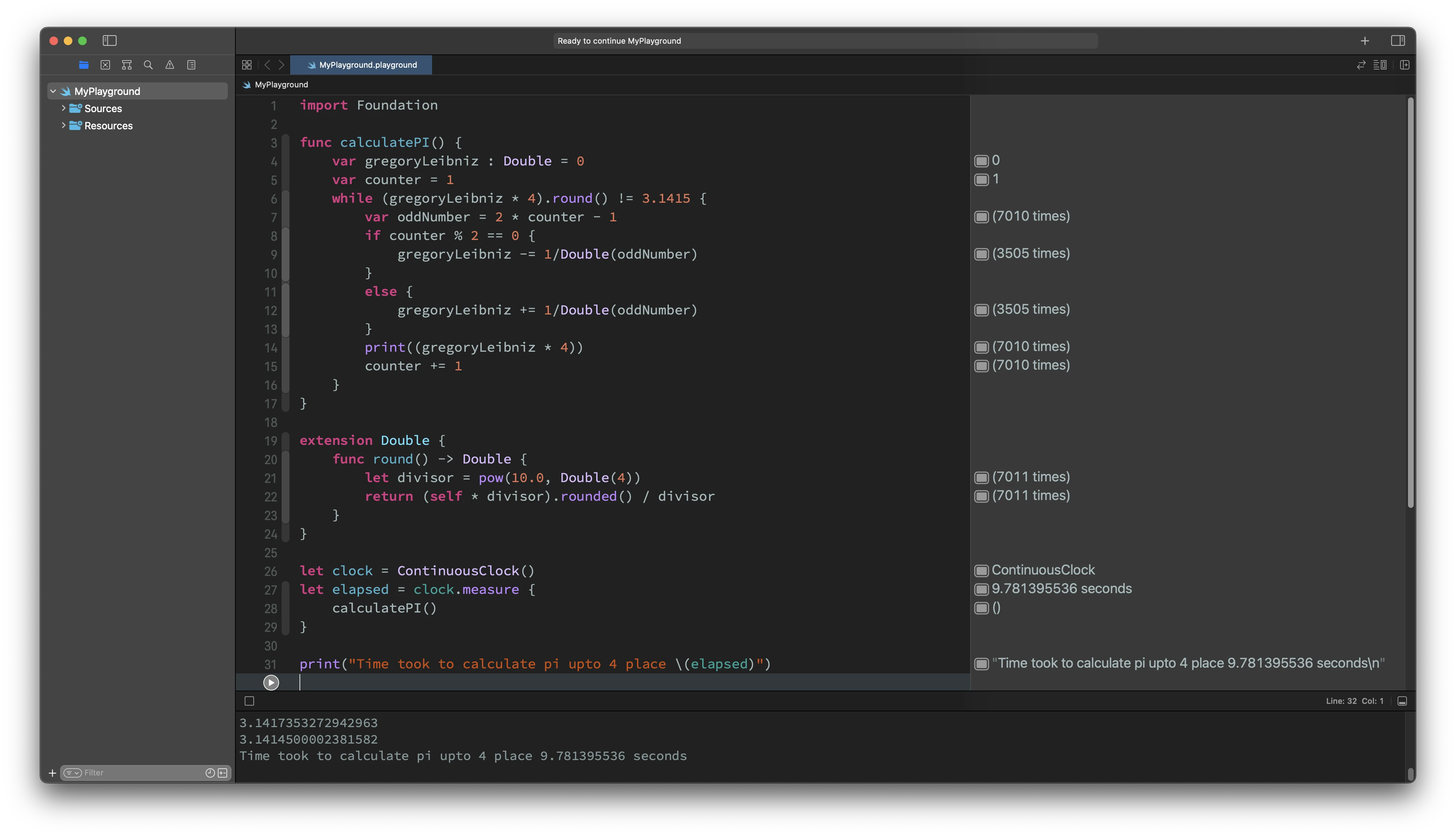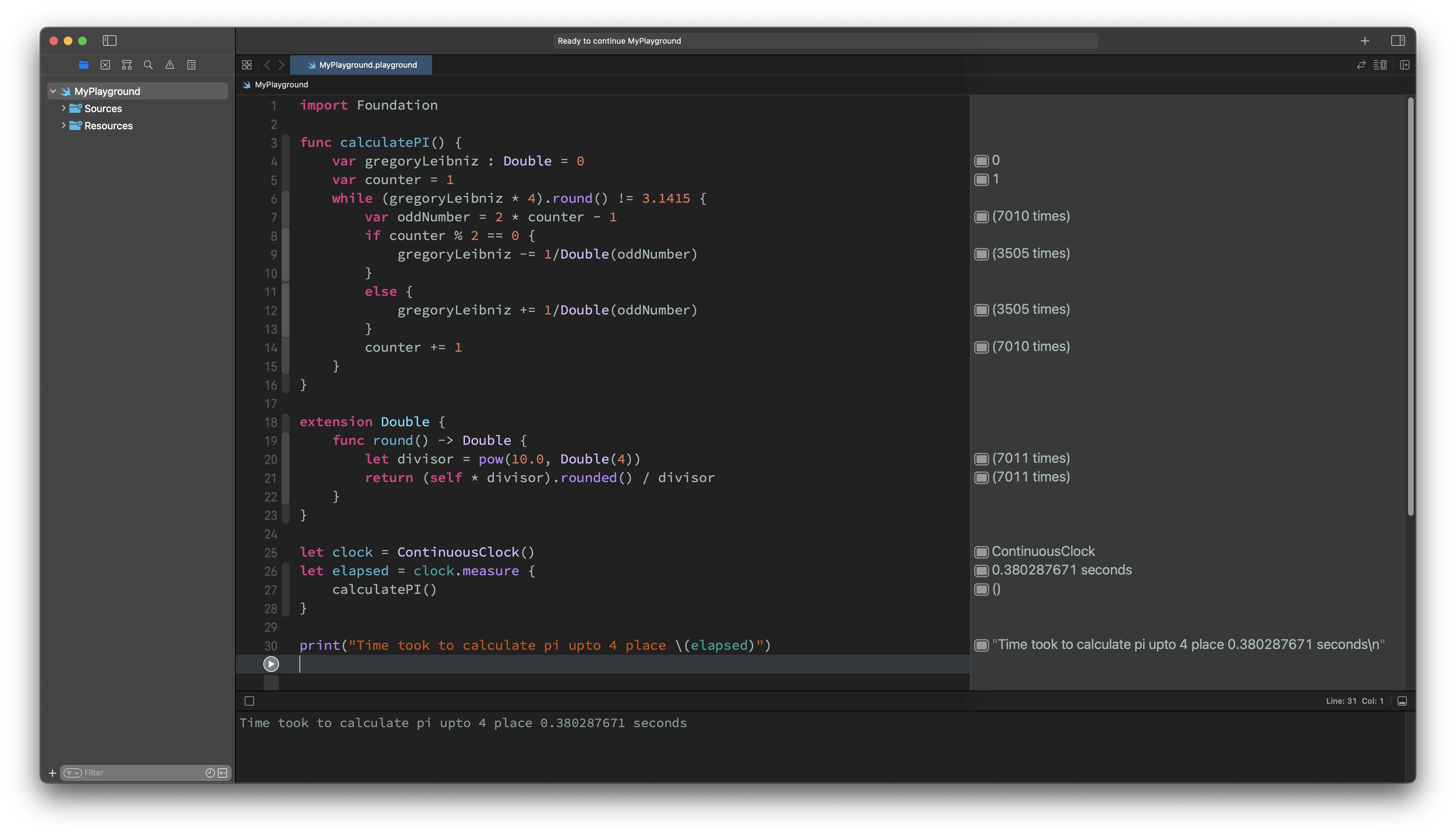Runtime code execution duration monitoring is crucial. Swift provides `ContinuousClock` to measures the time that always increments. The `ContinuousClock` acts like a stopwatch, measuring time relative to when a program starts or some other specific reference point. This is suitable for high resolution measurements of execution.

## 1. Measure function execution time

You can use `ContinuousClock` `measure(_:)` function to measure execution time for any function/code. You can use the `measure(_:)` function like this:

``````let clock = ContinuousClock()
let elapsed = clock.measure {
someWork()
}
print("Time: \(elapsed)")
``````

## 2. Measure asynchronous function execution time

If you want to measure an asynchronous function then you can use the asynchronous `measure(_:)` function like this:

``````let clock = ContinuousClock()
let elapsed = await clock.measure {
await someWork()
}
print("Time: \(elapsed)")
``````

## 3. Example

For example, let's calculate the value of PI up to 4 decimal places using the Leibniz formula.

``````import Foundation

func calculatePI() {
var gregoryLeibniz : Double = 0
var counter = 1
while (gregoryLeibniz * 4).round() != 3.1415 {
var oddNumber = 2 * counter - 1
if counter % 2 == 0 {
gregoryLeibniz -= 1/Double(oddNumber)
}
else {
gregoryLeibniz += 1/Double(oddNumber)
}
print((gregoryLeibniz * 4).round())
counter += 1
}
}

extension Double {
func round() -> Double {
let divisor = pow(10.0, Double(4))
return (self * divisor).rounded() / divisor
}
}

let clock = ContinuousClock()
let elapsed = clock.measure {
calculatePI()
}

print("Time took to calculate pi upto 4 place \(elapsed)")

``````

In the above example, inside the `calculatePI()` function there is a `print` statement. You can try to execute the same code with and without `print` statement to measure the impact of adding `print` statement in our code.

#### With print statement#### Without print statement